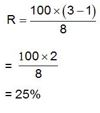# Simple Interest Questions

FACTS  AND  FORMULAE  FOR  SIMPLE  INTEREST  QUESTIONS

1. Principal: The money borrowed or lent out for a certain period is called the principal or the sum.

2. Interest: Extra money paid for using other's money is called interest

3. Simple Interest (S.I.) : If the interest on a sum borrowed for a certain period is reckoned uniformly, then it is called simple interest.

Let Principal = P, Rate = R% per annum (p.a.) and Time = T years. Then,

(i)  $S.I=\left(\frac{P×T×R}{100}\right)$

(ii)

Q:

A sum was put at simple interest at a certain rate for 10 years . Had it been put at 5% higher rate , it would have fetched Rs.600 more. What was the Sum?

 A) Rs.1200 B) Rs.1300 C) Rs.1400 D) Rs.1500

Explanation:

At 5% more rate, the increase in S.I for 10 years = Rs.600  (given)

So, at 5% more rate, the increase in SI for 1 year = 600/10 = Rs.60/-

i.e. Rs.60 is 5% of the invested sum

So, 1% of the invested sum = 60/5

Therefore, the invested sum = 60 × 100/5 = Rs.1200

29 17258
Q:

A father left a will of Rs.35 lakhs between his two daughters aged 8.5 and 16 such that they may get equal amounts when each of them reach the age of 21 years. The original amount of Rs.35 lakhs has been instructed to be invested at 10% p.a. simple interest. How much did the elder daughter get at the time of the will?

 A) 17.5 lakhs B) 21 lakhs C) 15 lakhs D) 20 lakhs

Explanation:

Let Rs.x be the amount that the elder daughter got at the time of the will. Therefore, the younger daughter got (3,500,000 - x).

The elder daughter’s money earns interest for (21 - 16) = 5 years @ 10% p.a simple interest.

The younger daughter’s money earns interest for (21 - 8.5) = 12.5 years @ 10% p.a simple interest.

As the sum of money that each of the daughters get when they are 21 is the same,

$x+5*10*x100=3,500,000-x+12.5*10*3,500,000-x100$

$x+50x=3,500,000-x+125100*3,500,000-125x100$

$2x+50x100+125x100=3,500,000*1+54$

$200x+50x+125x100=94*3,500,000$

=>

36 17104
Q:

A sum of money amounts to Rs. 9800 after 5 years and Rs. 12005 after 8 years at the same rate of simple interest. The rate of interest per annum is:

 A) 5% B) 8% C) 12% D) 15%

Explanation:

S.I. for 3 years = Rs. (12005 - 9800) = Rs. 2205.

S.I. for 5 years = Rs.$22053×5$= Rs.3675

Principle = Rs.(9800-3675) = Rs.6125

Hence, Rate = $100×36756125×5$ =12%

11 14481
Q:

A man borrowed Rs 24000 from two money lenders. For one loan, he paid 15% per annum and for the other 18% per annum. At the end of one year, he paid Rs 4050. How much did he borrow at each rate ?

 A) Rs.16000 B) Rs.12000 C) Rs.15000 D) Rs.13000

Explanation:

Let the sum at 15% be Rs x and that at 18% be Rs (24000 - x).

{(x * 15 * 1)/100 } + { [(24000 – x) * 18 * 1]/100 } = 4050

or 15 x + 432000 - 18x = 405000 or x = 9000.

Money borrowed at 15% = Rs 9000 .

Money borrowed at 18% = Rs 15000.

16 14419
Q:

A person takes a loan of Rs. 200 at 5% simple interest. He returns Rs.100 at the end of one year. In order clear his dues at the end of 2 years, he would pay :

 A) Rs. 100 B) Rs. 105 C) Rs. 115 D) Rs. 110

Explanation:

Amount to be paid = $Rs.100+200×5×1100+100×5×1100$= Rs. 115.

21 14159
Q:

How long will it take for a sum of money to grow from Rs.1250 to Rs.10,000, if it is invested at 12.5% p.a simple interest?

 A) 65years B) 56years C) 45years D) 57years

Explanation:

Simple interest is given by the formula SI = (pnr/100), where p is the principal, n is the numberof years for which it is invested, r is the rate of interest per annum

In this case, Rs. 1250 has become Rs.10,000.

Therefore, the interest earned = 10,000 – 1250 = 8750.

8750 = [(1250 x n x 12.5)/100]

=> n = 700 / 12.5 = 56 years.

18 13953
Q:

A Certain sum of money an amounts to Rs 2500 in a span Of 5 years and further to Rs.3000 in a span of 7 years at simple interest The sum is ?

 A) Rs. 1800 B) Rs. 2000 C) Rs. 1400 D) Rs. 1250

Explanation:

2500 in 5th year and 3000 in 7th year
So in between 2 years Rs. 500 is increased => for a year 500/2 = 250
So, per year it is increasing Rs.250 then in 5 years => 250 x 5 = 1250
Hence, the initial amount must be 2500 - 1250 = Rs. 1250

12 12851
Q:

A sum of money triples itself in 8 years simple interest. Find the rate of percent per annum?

 A) 30% B) 25% C) 22% D) 18%